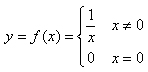# 7-1 计算 11+12+13+...+m

### 输入样例:

90


### 输出样例:

sum = 4040

### 代码：

m=int(input("请输入一个正整数m(20<=m<=100)"))
print(sum(list(range(11,m+1))))

# 7-2 计算分段函数### 输入样例1:

10


### 输出样例1:

f(10.0) = 0.1


### 输入样例2:

0


### 输出样例2:

f(0.0) = 0.0

### 代码：

def f(x):
if x!=0:
result=1/x
else:
result=0
return result
x=float(input())
print("{0:.1f}".format(f(x)))

# 7-3 阶梯电价

### 输入样例1:

10


### 输出样例1:

cost = 5.30


### 输入样例2:

100


### 输出样例2:

cost = 55.50

### 代码：

def ecost(x):
if x<=50:
cost=x*0.53
else:
cost=(x-50)*(0.53+0.05)+50*0.53
return cost
x=float(input("请输入月用电量:\n"))
print("{0:.2f}".format(ecost(x)))

# 7-4 特殊a串数列求和

### 输入样例：

2 3


### 输出样例：

s = 246

### 代码：

a,n=input().split()
num=[int(a*i) for i in range(1,int(n)+1)]
print("s=%d"%sum(num))

# 7-5 求奇数分之一序列前N项和

### 输入样例:

23


### 输出样例:

sum = 2.549541

### 代码：

n=int(input("请输入一个正整数:\n"))
seris=[1/i for i in range(1,2*(n+1)-1) if i%2==1]
print("sum=%.6f"%sum(seris))


# 7-6 求交错序列前N项和

### 输入样例:

5


### 输出样例:

0.917

### 代码：

n=int(input("请输入一个正整数:\n"))
seris=[i/(2*i-1) if i%2!=0 else -i/(2*i-1) for i in range(1,n+1)]
print("{:.3}".format(sum(seris)))

# 7-7 产生每位数字相同的n位数

### 输入样例1:

  1,  5


### 输出样例1:

11111


### 输入样例2:

        3  ,4


### 输出样例2:

3333

### 代码：

line=input("请输入正整数A(1=<A<=9)和正整数B(1<=B<=10):\n").replace(' ','')
A,B=line.split(",")
print(A*int(B))

# 7-8 转换函数使用

### 输入样例:

45,8


### 输出样例:

37

### 代码：

n,m=input().split(',')
print(int(n,int(m)))

# 7-9 比较大小

### 输入样例:

4 2 8


### 输出样例:

2->4->8

### 代码：

num=list(map(int,input().split()))
num.sort()
print("%d->%d->%d"%(num,num,num))

# 7-10 输出华氏-摄氏温度转换表

### 输入样例1:

32 35


### 输出样例1:

fahr celsius
32   0.0
34   1.1


### 输入样例2:

40 30


### 输出样例2:

Invalid.

### 代码：

lower,upper=map(int,(input("请输入2个正整数lower和upper(lower<=upper<=100):\n").split()))
if lower<=upper and upper<=100:
for i in range(lower,upper,2):
c=5*(i-32)/9
print("%6d%6.1f"%(i,c))
else:
print("Invalid")

# 7-11 求平方与倒数序列的部分和

### 输入样例:

5 10


### 输出样例:

sum = 355.845635

### 代码：

m,n=map(int,input("请输入两个正整数m和n（m<=n)\n").split())
seris1=[i**2 for i in range(m,n+1)]
seris2=[1/i for i in range(m,n+1)]
print("sum=%.6f"%(sum(seris1)+sum(seris2)))

# 7-12 输出三角形面积和周长

### 输出格式：

area = 面积; perimeter = 周长


These sides do not correspond to a valid triangle


### 输入样例1：

5 5 3


### 输出样例1：

area = 7.15; perimeter = 13.00


### 输入样例2：

1 4 1


### 输出样例2：

These sides do not correspond to a valid triangle

### 代码：

import math
a,b,c=map(int,input("请输入三角形的三条边a、b和c\n").split())
if (a+b)>c and (a+c)>b and (b+c)>a:
s=(a+b+c)/2
area=math.sqrt(s*(s-a)*(s-b)*(s-c))
per=a+b+c
print("area = %.2f;perimeter = %.2f"%(area,per))
else:
print("These sides do not correspond to a valid triangle")

# 7-13 分段计算居民水费

### 输入样例1：

12


### 输出样例1：

16.00


### 输入样例2：

16


### 输出样例2：

22.50

### 代码：

x=float(input("请输入一个非负数:\n"))
while True:
if x>=0:
if x<=15:
y=4*x/3
else:
y=2.5*x-17.5
print("%.2f"%y)
break
else:
x=float(input("请输入一个非负数:\n"))

# 7-14 求整数段和

### 输入样例：

-3 8


### 输出样例：

   -3   -2   -1    0    1
2    3    4    5    6
7    8
Sum = 30

### 代码：

A,B=map(int,(input("请输入两个整数(-100<=A<=B<=100):\n").split()))
if A>=-100 and B<=100 and A<=B:
cnt=0
for i in range(A,B+1):
if i!=B:
cnt=cnt+1
if cnt<5:
print("%5d"%i,end='')
else:
print("%5d"%i)
cnt=0
else:

print("sum = %d"%sum(list(range(A,B+1))))

03-2683408-031577
05-151万+
02-213409
03-291万+
11-012570
10-233981
04-18585
10-162158